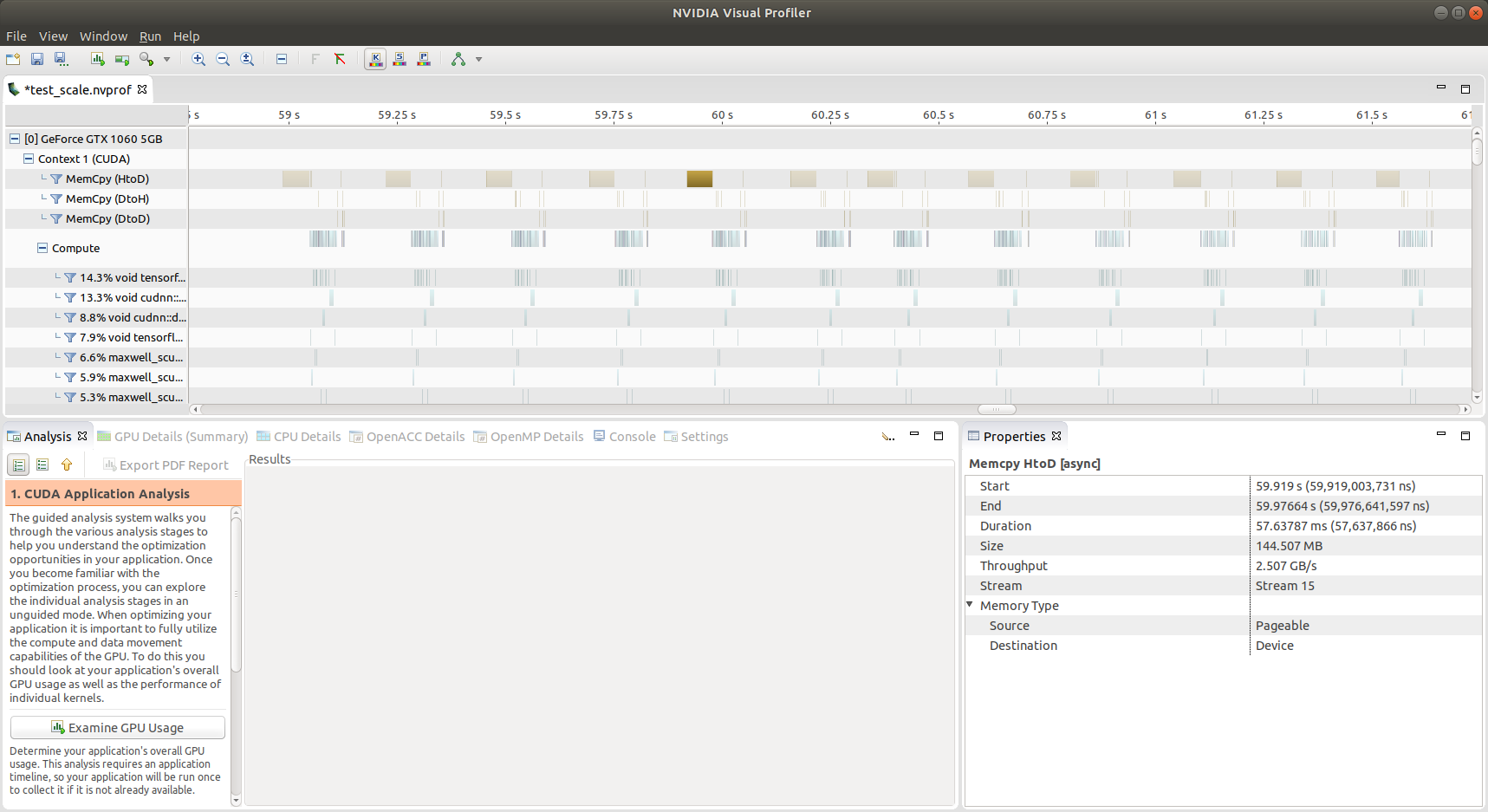18 August 2021

# OpenAI baselines ScaledFloatFrame fix - reduces training time in half

by Mathieu Poliquin

Almost 3 years ago I found a rather huge performance bug in OpenAI’s baselines that cut training FPS by half depending on the use case as well as cause some accuracy issues since the image input data was not correct. You can find the github issue here:

Since OpenAI have put baseline project in maintance mode and that they seem to not process Pull Requests anymnore I made a fork of baselines with the fix:

### Explaination

The issue was that the input image to the model was normalized two times. Once on the CPU side via the ScaledFloatFrame wrapper and once on the GPU with Tensorflow. Which caused some obvious accurary problems since the input data to the data was not correct as well having a CPU overhead and PCIE bus data transfers 4x as large (going from 8 bit color buffers to floating point buffers)

You can see in the Nvidia visual profiler pic below how the data transfers take a huge chunk of time:These where the places in the code where scaling is applied: from retro_wrappers.py

``````def wrap_deepmind_retro(env, scale=False, frame_stack=4):
"""
Configure environment for retro games, using config similar to DeepMind-style Atari in wrap_deepmind
"""
env = WarpFrame(env)
env = ClipRewardEnv(env)
if frame_stack > 1:
env = FrameStack(env, frame_stack)
if scale:
env = ScaledFloatFrame(env)
return env
``````

from models.py

``````def nature_cnn(unscaled_images, **conv_kwargs):
"""
CNN from Nature paper.
"""
scaled_images = tf.cast(unscaled_images, tf.float32) / 255.
activ = tf.nn.relu
h = activ(conv(scaled_images, 'c1', nf=32, rf=8, stride=4, init_scale=np.sqrt(2),
**conv_kwargs))
h2 = activ(conv(h, 'c2', nf=64, rf=4, stride=2, init_scale=np.sqrt(2), **conv_kwargs))
h3 = activ(conv(h2, 'c3', nf=64, rf=3, stride=1, init_scale=np.sqrt(2), **conv_kwargs))
h3 = conv_to_fc(h3)
return activ(fc(h3, 'fc1', nh=512, init_scale=np.sqrt(2)))
``````

I made a video that shows the fix with Pong-Atari2600:

tags: OpenAI - baselines - retro - ScaledFloatFrame - performance - fps